Next: Conditioning the gradient Up: ITERATIVE METHODS Previous: ITERATIVE METHODS

## Method of random directions and steepest descent

Let us minimize the sum of the squares of the components of the residual vector given by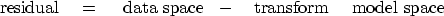(30)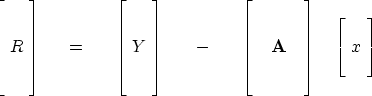(31)
Fourier-transformed variables are often capitalized. Here we capitalize vectors transformed by the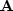matrix. A matrix such asis denoted by boldface print.

A contour plot is based on an altitude function of space. The altitude is the dot product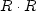.By finding the lowest altitude we are driving the residual vector R as close as we can to zero. If the residual vector R reaches zero, then we have solved the simultaneous equations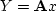.In a two-dimensional world the vector x has two components, (x1 , x2 ). A contour is a curve of constantin (x1 , x2 )-space. These contours have a statistical interpretation as contours of uncertainty in (x1 , x2 ), given measurement errors in Y.

Starting from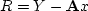, let us see how a random search direction can be used to try to reduce the residual. Let g be an abstract vector with the same number of components as the solution x, and let g contain arbitrary or random numbers. Let us add an unknown quantityof vector g to vector x, thereby changing x to.The new residual R+dR becomes(32) (33) (34)
We seek to minimize the dot product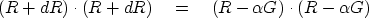(35)
Setting to zero the derivative with respect togives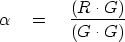(36)
Geometrically and algebraically the new residual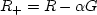is perpendicular to the fitting function'' G. (We confirm this by substitution leading toIn practice, random directions are rarely used. It is more common to use the gradient vector. Notice also that a vector of the size of x is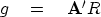(37)
Notice also that this vector can be found by taking the gradient of the size of the residuals: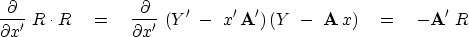(38)
Descending by use of the gradient vector is called the method of steepest descent."Next: Conditioning the gradient Up: ITERATIVE METHODS Previous: ITERATIVE METHODS
Stanford Exploration Project
10/21/1998